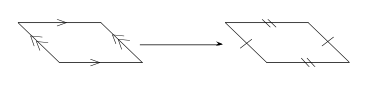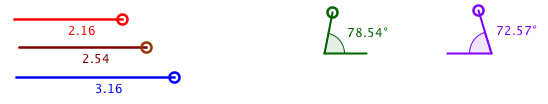Back to Teaching Proof

# Proof in High School Geometryby Henri Picciotto

I worked at the Urban School from 1981 to 2013, and helped develop much of the curriculum and pedagogy of the math department. For an overview of the Urban School math offerings when I was there, click here.

This article is a quick summary of Urban's Math 2 course back then, with an emphasis on the approach to proof. The course evolves from year to year, and may have changed substantially since then.

We use much material from my Geometry Labs book throughout the course. This work does not emphasize formal proof, but it does lay the foundation for it through discussion-generating activities.

We also use problems from a variety of books. My favorite by far is Geometry: A Guided Inquiry by Chakerian, Crabill and Stein. This is the book that had the greatest impact on my approach to teaching high school math. We also use a few problems from Michael Serra's Discovering Geometry and a few from Harold Jacobs' Geometry, Third Edition.

We start with much work on the inscribed angle theorem and the Pythagorean theorem, plus some algebra and an intro to computer programming. In the second quarter of the course, we introduce congruent triangles. A foundational activity is "Non-Congruent Triangles", from Geometry Labs. (However we use patty paper to copy angles, not compass and straightedge. The latter is too complicated and not worth the time it takes to teach it.) We follow that up by having students do the usual very basic proofs using those postulates, without requiring any particular format.

Around the same time, we introduce interactive geometry software (we have used Cabri, but the same can be accomplished with Geometer's Sketchpad or GeoGebra.) One early lesson in that environment asks students to create triangles using SSS, SAS, and so on. This is a complement to the "non-congruent triangles" lesson and it helps to develop the students' understanding of the congruence postulates, to get to the bottom of why AAA and SSA do not guarantee congruency, and to discover the triangle inequality. Here is a screen shot of the GeoGebra version of this activity. The lengths and angles can of course be manipulated dynamically.If you create a GSP sketch or Cabri figure of this,
please send it to me and I'll post it here.

In the second half of the course, among other things, we do these three units:
Proof